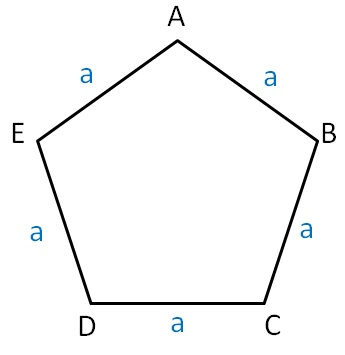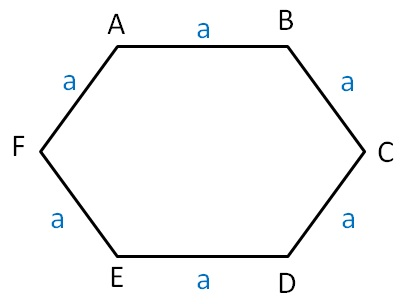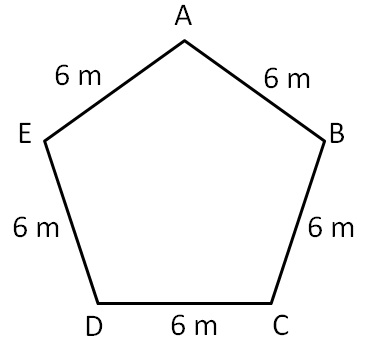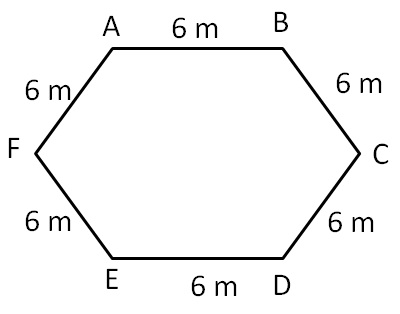Perimeter of Pentagon and Hexagon

Chapter 10 Class 6 Mensuration
Concept wise

We know that

Pentagon has 5 sidesHere we talk about Regular pentagon

Regular pentagon has all sides equal

∴  AB = BC = CD = DE = AE = a

Now,

Perimeter = Sum of all sides

= AB + BC + CD + DE + AE

= a + a + a + a + a

= 5a

#### Hexagon

We know that

Hexagon has 6 sidesHere we talk about Regular hexagon

Regular hexagon has all sides equal

∴  AB = BC = CD = DE = EF = FA = a

Now,

Perimeter = Sum of all sides

= AB + BC + CD + DE + EF + FA

= a + a + a + a + a + a

= 6a

#### An example of regular pentagon is,Here,

AB = BC = CD = DE = AE = a = 6m

Perimeter of regular pentagon = 5a

= 5 × 6

= 30 m

#### And that of a regular hexagon is,Here,

AB = BC = CD = DE = EF = AF = a = 6m

Perimeter of regular hexagon = 6a

= 6 × 6

= 36 m

Learn in your speed, with individual attention - Teachoo Maths 1-on-1 Class